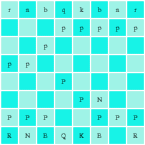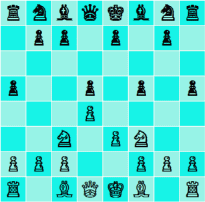# Toledo Javascript Chess Game

Around January 2007 I remembered the similarities between C and Javascript, and I was wondering if it would be possible to do a translation of my recent winning entry (19th IOCCC, Most Portable Chess Set), I did it on an afternoon and the result is the current world's smallest chess program in Javascript, check also my Tiny Chess for JS1K.
Play it online:
You can select the promotion piece using the control. Some browsers will show you a message of script being too slow, please indicate that you DON'T want to stop the script.
See it on action, to play click piece to move and then click destination square:
If you want to play with black's pieces, click this to force a move, you will need to click every time after you do a move.

## The source code

Most of the code is a resemblance of the original code, except for the pointers translated to array-access. Recently I updated the core with code from Toledo Nanochess Nov/20/2009.
Play level is fixed at 3-ply depth, you can modify it (search on the source code for /*ply*/), be careful as every extra ply is an order of magnitude more slow.
A brief description of the inner workings of this code can be found on the page of Toledo Chess 1 or check my Toledo Nanochess book.
```<script>//(c)2009 Oscar Toledo G.
var B,i,y,u,b,I=[],G=120,x=10,z=15,M=1e4,l=[5,3,4,6,2,4,3,5,1,1,1,1,1,1,1,1,9,9
,9,9,9,9,9,9,13,11,12,14,10,12,11,13,0,99,0,306,297,495,846,-1,0,1,2,2,1,0,-1,-
1,1,-10,10,-11,-9,9,11,10,20,-9,-11,-10,-20,-21,-19,-12,-8,8,12,19,21];function
X(w,c,h,e,S,s){var t,o,L,E,d,O=e,N=-M*M,K=78-h<<x,p,g,n,m,A,q,r,C,J,a=y?-x:x;
y^=8;G++;d=w||s&&s>=h&&X(0,0,0,21,0,0)>M;do{if(o=I[p=O]){q=o&z^y;if(q<7){A=q--&
2?8:4;C=o-9&z?[53,47,61,51,47,47][q]:57;do{r=I[p+=l[C]];if(!w|p==w){g=q|p+a-S?0
:S;if(!r&(!!q|A<3||!!g)||(r+1&z^y)>9&&q|A>2){if(m=!(r-2&7))return y^=8,I[G--]=
O,K;J=n=o&z;E=I[p-a]&z;t=q|E-7?n:(n+=2,6^y);while(n<=t){L=r?l[r&7|32]-h-q:0;if(
s)L+=(1-q?l[(p-p%x)/x+37]-l[(O-O%x)/x+37]+l[p%x+38]*(q?1:2)-l[O%x+38]+(o&16)/2:
!!m*9)+(!q?!(I[p-1]^n)+!(I[p+1]^n)+l[n&7|32]-99+!!g*99+(A<2):0)+!(E^y^9);if(s>h
||1<s&s==h&&L>z|d){I[p]=n,I[O]=m?(I[g]=I[m],I[m]=0):g?I[g]=0:0;L-=X(s>h|d?0:p,L
-N,h+1,I[G+1],J=q|A>1?0:p,s);if(!(h||s-1|B-O|i-n|p-b|L<-M))return W(),G--,u=J;
J=q-1|A<7||m||!s|d|r|o<z||X(0,0,0,21,0,0)>M;I[O]=o;I[p]=r;m?(I[m]=I[g],I[g]=0):
g?I[g]=9^y:0;}if(L>N||s>1&&L==N&&!h&&Math.random()<.5){I[G]=O;if(s>1){if(h&&c-L
<0)return y^=8,G--,L;if(!h)i=n,B=O,b=p;}N=L;}n+=J||(g=p,m=p<O?g-3:g+2,I[m]<z|I[
m+O-p]||I[p+=p-O])?1:0;}}}}while(!r&q>2||(p=O,q|A>2|o>z&!r&&++C*--A));}}}while(
++O>98?O=20:e-O);return y^=8,G--,N+M*M&&N>-K+1924|d?N:0;}B=i=y=u=0;while(B++<
120)I[B-1]=B%x?B/x%x<2|B%x<2?7:B/x&4?0:l[i++]|16:7;for(a=
"<table cellspacing=0 align=center>",i=18;i<100;a+=++i%10-9?
"<th width=40 height=40 onclick=Y("+i+") style='border:2px solid #aae' id=o"+i+
" bgcolor=#"+(i*.9&1?"9090d0>":"c0c0ff>"):(i++,"<tr>"));
a+="<th colspan=8><select id=t><option>Q<option>R<option>B";
document.write(a+"<option>N</select></table>");
function W(){B=b;for(p=21;p<99;++p)if(q=document.getElementById("o"+p)){q.
innerHTML="<img width=40 src="+(I[p]&z)+".gif>";q.
style.borderColor=p==B?"#ff0":"#aae";}}W();
function Y(s){i=(I[s]^y)&z;if(i>8){b=s;W();}else if(B&&i<9){b=s;i=I[B]&z;if((i&
7)==1&(b<29|b>90))i=14-document.getElementById("t").selectedIndex^y;X(0,0,0,21,
u,1);if(y)setTimeout("X(0,0,0,21,u,2/*ply*/),X(0,0,0,21,u,1)",250);}}
</script>```

## Source code forks

This Javascript chess game has currently several forks, same core but different graphical interfaces:

## Tiny chess for the JS1K contest

The JS1K contest started on Sunday, August 1, 2010, organized by Peter van der Zee with Thomas Fuchs (author of Scriptaculous), Remy Sharp, Patrick H. Lauke and Christopher Williams as judges, all expert Javascript programmers. The contest's objective was simple, the participants should create the most impressive Javascript program in 1024 bytes, that is 1 kilobyte, without using any external file. The contest period closed on September 10.
I had news of the contest in August 8 and came to my mind the challenge of writing a chess in 1K of Javascript, so based on my 2.2K Javascript chess in six hours I managed to crunch it to 1K. I had to remove castling, en passant and limit promotion to queen, leaving a simple chessboard with letters for chessmen, click in origin square and target square to move pieces.
Next day I sent it to the contest, you can still play it online as the entry #226. Take note that the 1018 octets of my Javascript code include an artificial intelligence and also the user's interface and chessboard visualization. Not an easy thing as noted by several people.First version of Tiny Chess
Two hours after publication, the enthusiast Laxminarayan Kamath sent me an e-mail suggesting me to put Unicode graphics and an optimization tip that I would use latter. On Wednesday 11 in other six hours of work I cutted more than 50 bytes, making enough space to put Unicode graphics for the chess pieces, I sized up the chessboard for comfortable visualization and also illuminated clicked square. Same day I sent this update to the contest (it obtained the number 298), and the graphics surprised to the public.
Some days after Spaniard Román Cortés kindly shared with me optimization tips for saving bytes, at same time I rewrote the artificial intelligence to save space and with the extra space introduced variety in gameplay (Math.random) also instant update of chessboard on final click. This was the third update (entry 435) and got excellent and multitude of comments, hundreds of mentions in Twitter and thousands of visitors from United States, Spanish, Chinese, Japanese, Russian, French and German forums and blogs. I've estimated that this version was played by at least 25,000 people around the world, this number was greatly surpassed by my last version played by an estimate of 100,000 people.The third version of Tiny Chess
An anonymous German reported that was easy to force my program to fool's mate, I realized that an extra «intelligence» would solve the problem and also I did illumination of last moved piece. This was the fourth update (entry 699).
Casually same day that I did the update, I received an e-mail from English Matt Round, he informed me of his article about a game of my chess versus the legendary 1K ZX81 Chess, and my chess drew instead of giving mate. I knew immediately it could be enhanced, so I was on the task again, was very difficult to «crunch» these last bytes (here I finally used Kamath's optimization tip), I did it and was my last update (entry 750) before contest closed.
And it won!, on September 25, 2010, Thomas Fuchs and Remy Sharp demonstrated the winning JS1K entries at Berlin, Germany, during the jsConf.eu conference, my Tiny Chess got 2nd place, triggering a wave of mentions.
Here is the definitive version of my Javascript Chess in 1023 bytes, note that the original version was written in a single line that has to be divided to put it here, also this is pure Javascript, must be invocated by HTML markers such as this <body> <script src="tiny_chess.js"> </body>
```// Tiny Chess (c)2010 Óscar Toledo G.
for(B=i=y=u=b=i=5-5,x=10,I=[],l=[];B++<304;
I[B-1]=B%x?B/x%x<2|B%x<2?7:B/x&4?0:l[i++]=("ECDFBDCEAAAAAAAA"+
"IIIIIIIIMKLNJLKM@G@TSb~?A6J57IKJT576,+-48HLSUmgukgg OJNMLK  IDHGFE")
.charCodeAt(y++)-64:7);function X(c,h,e,s){c^=8;for(var o,S,C,A,R,T,G,d=e&&X(c,0
)>1e4,n,N=-1e8,O=20,K=78-h<<9;++O<99;)if((o=I[T=O])&&(G=o^c)<7){A=G--&2?8:4;C=
o-9?l[61+G]:49;do if(!(R=I[T+=l[C]])&&!!G|A<3||(R+1^c)>9&&G|A>2){if(!(R-2&7)
)return K;n=G|(c?T>29:T<91)?o:6^c;S=(R&&l[R&7|32]*2-h-G)+(n-o?110:!G&&(A<2)+1);
if(e>h||1<e&e==h&&S>2|d){I[T]=n;I[O]=0;S-=X(c,h+1,e,S-N);if(!(h||e-1|B-O|T-b|S<
-1e4))return W(),c&&setTimeout("X(8,0,2),X(8,0,1)",75);I[O]=o;I[T]=R}if(S>N||!h
&S==N&&Math.random()<.5)if(N=S,e>1)if(h?s-S<0:(B=O,b=T,0))break}while(!R&G>2||(
T=O,(G||A>2|(c?O>78:O<41)&!R)&&++C*--A))}return-K+768<N|d&&N}function W(){i=
"<table>";for(u=18;u<99;document.body.innerHTML=i+=++u%x-9?
"<th width=60 height=60 onclick='I[b="+u+
"]>8?W():X(0,0,1)'style='font-size:50px'bgcolor=#"+(u-B?u*.9&1||9:"d")+
"0f0e0>&#"+(I[u]?9808+l[67+I[u]]:160):u++&&"<tr>")B=b}W()```
I managed to get a version with castling and en passant in 1024 bytes (yes, it is possible!), but the visualization stood in black and white, also the gameplay quality is bad, works as a prototype, but was not nice enough for the contest. It was archived (although I can publish it here if there are interesting offers :)), in preference to an expanded version of my final entry with castling and en passant, here is that version in 1251 bytes.
```// Chess (c)2010 Óscar Toledo G.
for(B=i=y=u=b=i=5-5,x=10,z=15,I=[],l=[];l[B]=("ustvrtsuqqqqqqqq"+
"yyyyyyyy}{|~z|{}@G@TSb~?A6J57IKJT576,+-48HLSUmgukgg OJNMLK  IDHGFE")
.charCodeAt(B)-64,B++<120;I[B-1]=B%x?B/x%x<2|B%x<2?7:B/x&4?0:l[u++]:7);
function X(c,h,e,S,s){c^=8;for(var T,o,L,E,D,O=20,G,N=-1e8,n,g,d=S&&X(c,0)>1e4,
C,R,A,K=78-h<<9,a=c?x:-x;++O<99;)if((o=I[T=O])&&(G=o&z^c)<7){A=G--&2?8:4;C=9-o&
z?l[61+G]:49;do{R=I[T+=l[C]];g=D=G|T+a-e?0:e;if(!R&&(G||A<3||g)||(1+R&z^c)>9&&G
|A>2){if(!(2-R&7))return K;for(E=n=G|I[T-a]-7?o&z:6^c;E;E=!E&&!d&&!(g=T,D=T<O?g
-3:g+2,I[D]<z|I[D+O-T]|I[T+=T-O])){L=(R&&l[R&7|32]*2-h-G)+(G?0:n-o&z?110:(D&&14
)+(A<2)+1);if(S>h||1<S&S==h&&L>2|d){I[T]=n,I[g]=I[D],I[O]=D?I[D]=0:0;L-=X(c,h+1
,E=G|A>1?0:T,S,L-N);if(!(h||S-1|B-O|T-b|L<-1e4))return W(y=E),c&&setTimeout(
"X(8,0,y,2),X(8,0,y,1)",75);E=1-G|A<7|D|!S|R|o<z||X(c,0)>1e4;I[O]=o;I[T]=R;I[D
]=I[g];D?I[g]=G?0:9^c:0}if(L>N||!h&L==N&&Math.random()<.5)if(N=L,S>1)if(h?s-L<0
:(B=O,b=T,0))return N}}}while(!R&G>2||(T=O,G|A>2|z<o&!R&&++C*--A))}return-K+
768<N|d&&N}function W(){i="<table>";for(u=18;u<98;document.body.innerHTML=i+=++
u%x-9?"<th width=60 height=60 onclick='I[b="+u+
"]&8?W():X(0,0,y,1)'style='font-size:50px'bgcolor=#"+(u-B?u*.9&1||9:"d")+
"0f0e0>&#"+(I[u]&15?9808+l[67+(I[u]&15)]:160)+";":u++&&"<tr>")B=b}W()```